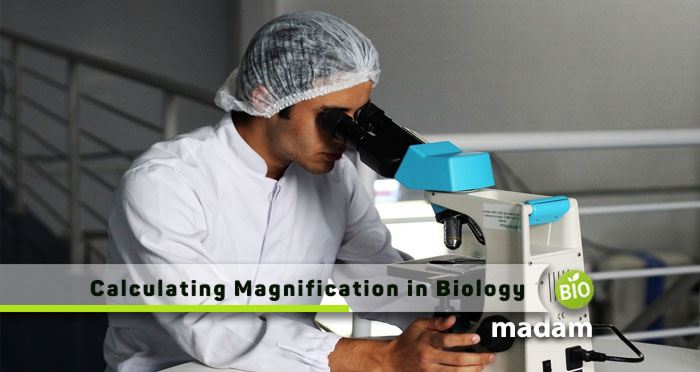# How to Calculate Magnification in BiologyBiology subject deal with a lot of technicalities and research. A biologist needs to be perfect in his investigations from every angle so that the studies and results are realistic and accurate.

A biologist investigates different animals, plants, microorganisms, cells, tissues, etc., and therefore needs to see the object properly, and for that magnification plays a significant role.

In this article, I will be discussing what magnification is, how it is essential in general and in biology, along with various methods to calculate magnification so that the researches are precise and accurate.

## What is Magnification

Magnification is a process that helps in enlarging the apparent size of an object and not the physical dimension. The process helps better visualize an object under study and see every minor detail for proper research and analysis.

## Importance of Magnification

Magnification holds very significant importance in various industries and professions. This phenomenon has helped in accuracy and better results by making insights of an object clear and easier to visualize.

Magnification has not only helped biologists, but it also helps coin collectors in analyzing coins, electricians in soldering of thin and complicated wiring of circuit and motherboard, and physics experts analyzing thin wires and structure of machines more clearly.

## Uses of Magnification in Biology

Biologists very commonly use magnification to analyze specimens and write their findings. This powerful tool helps see a bigger picture of tiny cells and objects and explore What’s inside it, the structure, and the functioning of the specimen.

A biologist works with animal plants and humans and researches them and helps in the medical profession. These experts need to visualize everything perfectly to be able to conclude things that are accurate and helpful for animals, humans, or plants.

Magnification has helped biologists a lot in improving their analyses and research skills and dig into objects more deeply and find more interesting facts about their specimens.

## How to Calculate Magnification in Biology

There are few methods to calculate magnification in biology. These are discussed below:

### By Equation

The simplest method to calculate the magnification of any object is by using the following equation:

Magnification = length of the image / actual size of the object

This equation helps identify how to enlarge the size of the object is from its actual size and what are the dimensions on which the researcher has researched. The equation can also be rearranged to help a biologist determine the actual size of an object under study if its magnification and length are known.

The actual size of an object = length of the object/magnification

A researcher sometimes needs to calculate the magnification or actual size of an object. In these cases, this equation helps best in knowing the mathematical values associated with the research.

### By Using Scale

Scale bar is another method to calculate the magnification of an object. In this method, the researcher needs to calculate the length of an object before and after magnification and determine how much the apparent size has increased during the process of magnification

The formula to calculate magnification using this method is similar to the way mentioned above and can be written as:

Magnification = scale bar image/ actual scale bar length

The two aforementioned methods are now not used frequently because of innovation in tools and technologies for biologists and researchers. Still, biologists should know all the methods to calculate magnification as they might need to work on unfavorable situations as well.

### By using Microscope

One of the most commonly used methods to calculate magnification nowadays is by using microscopes. There are two types of microscopes, compound microscope, and digital microscope, both of which can now help in not only magnifying the object but calculate the magnification also.

The microscopes now have features with which they can calculate the magnification, length, size, and all other dimensions of the object under study without any extra effort. The calculations are accurate and contain fewer chances of errors which helps biologists in improving their research.

## The Most Appropriate Method to Calculate Magnification

No Doubt, the fact is very clear that the most appropriate method to calculate magnification is by using microscopes. This approach is easiest, convenient, and effortless to get an accurate calculation of magnification by spending less time and doing no work for that.

Every biologist needs a microscope to analyze the specimen so why not buy such a microscope that can calculate magnification as well to make research and investigation more easier and reliable.

## Conclusion

Magnification is a very helpful technique for biologists to make research easier and accurate. Microscopes are made to help in the magnification of objects and therefore, this is the most appropriate method to calculate magnification.

There are economical microscopes that provide magnification calculation as well so you should check out those for sure.